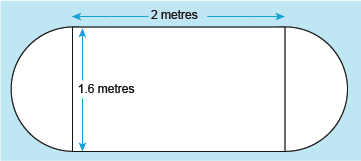Succeed with maths – Part 2

Start this free course now. Just create an account and sign in. Enrol and complete the course for a free statement of participation or digital badge if available.

Free course

# 2.3 Perimeters of mixed shapes

Suppose the shape that you wanted to determine the perimeter of is as shown in Figure 15.Figure 15 A shape formed of a rectangle and two semicircles

Start by noticing that the shape is formed of one rectangle and two semicircles. The distance around the two semicircles combined is the same as around a circle with a diameter of 1.6 m. So, calculating the circumference of a circle with this diameter will give us part of the answer. To find the total distance, or perimeter, you then just need to add in the lengths of the longest edges of the rectangle. This gives us the following calculation:

So, if you are faced with a complex shape, look for ways to split these up to help you calculate the perimeter.

Another property of a shape that can be useful to know is the area, or space that it occupies. This is slightly more complicated than the perimeter, but for regular shapes there are a set of formulas to help with this, as you will see in the next section.